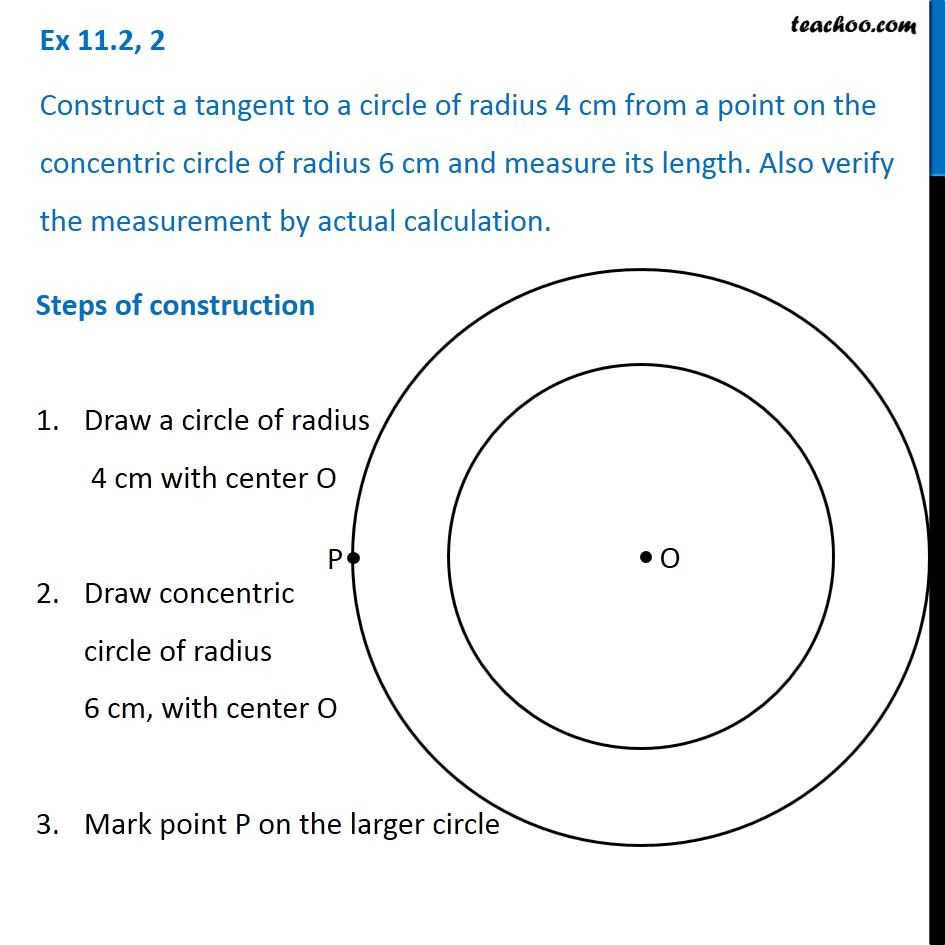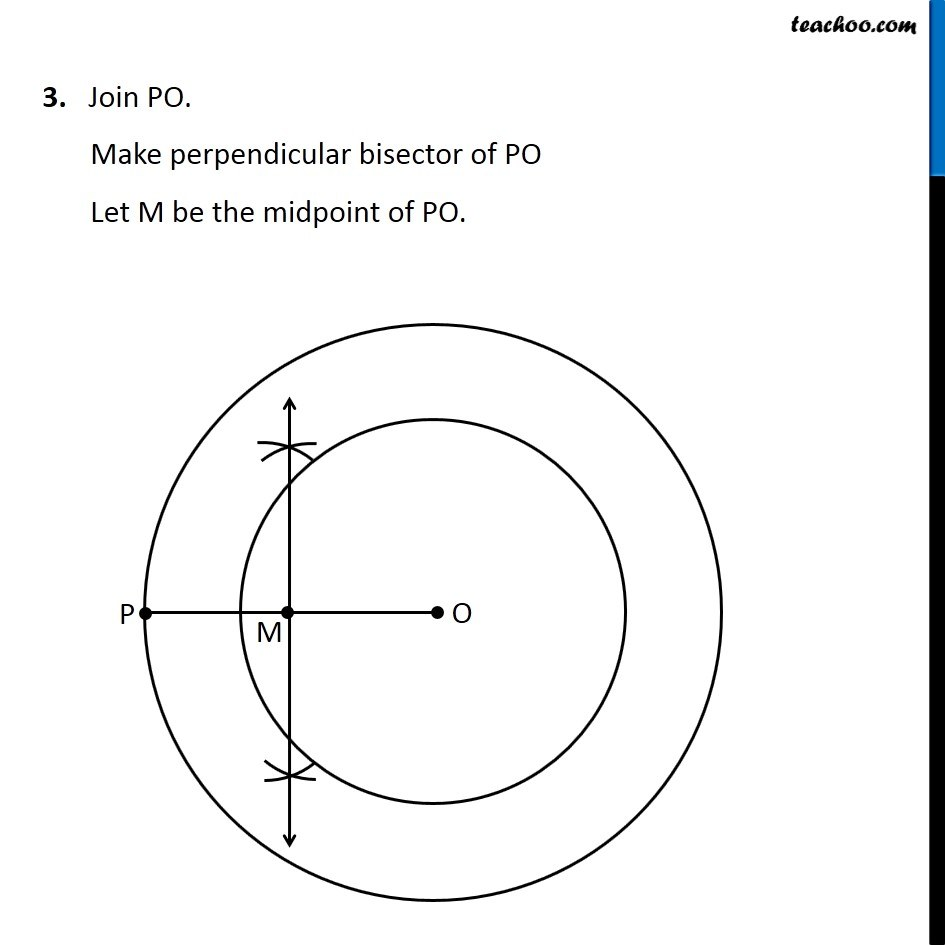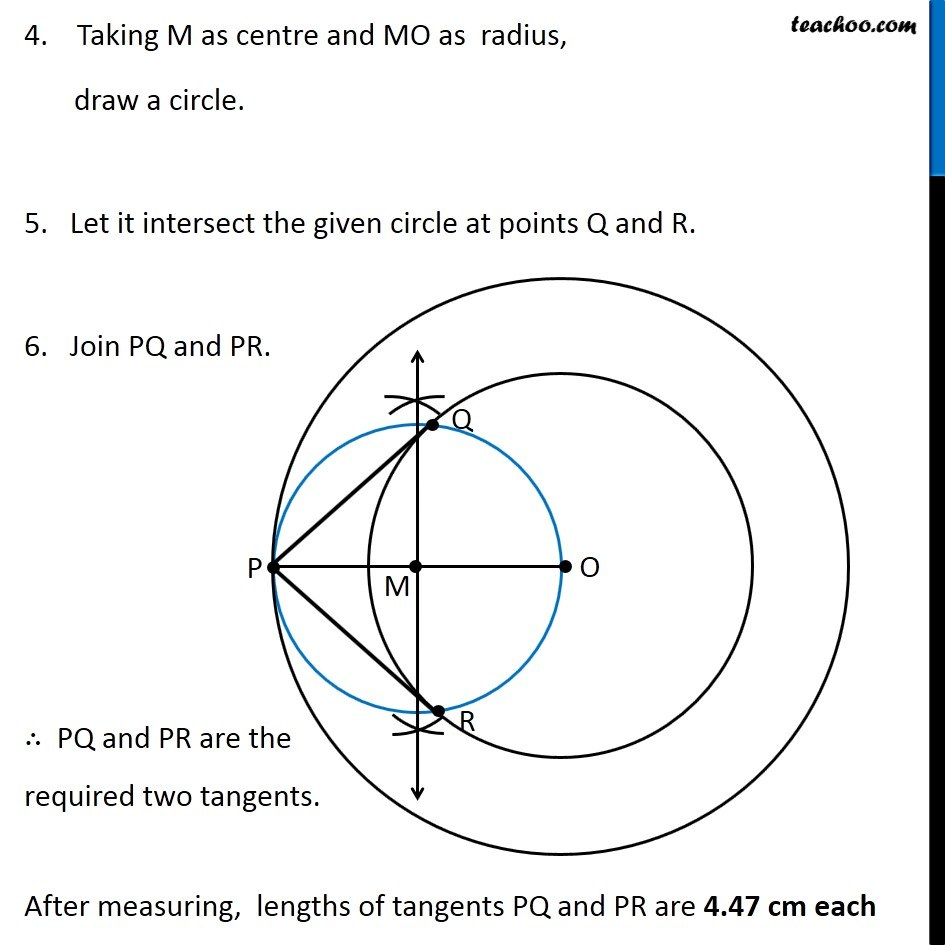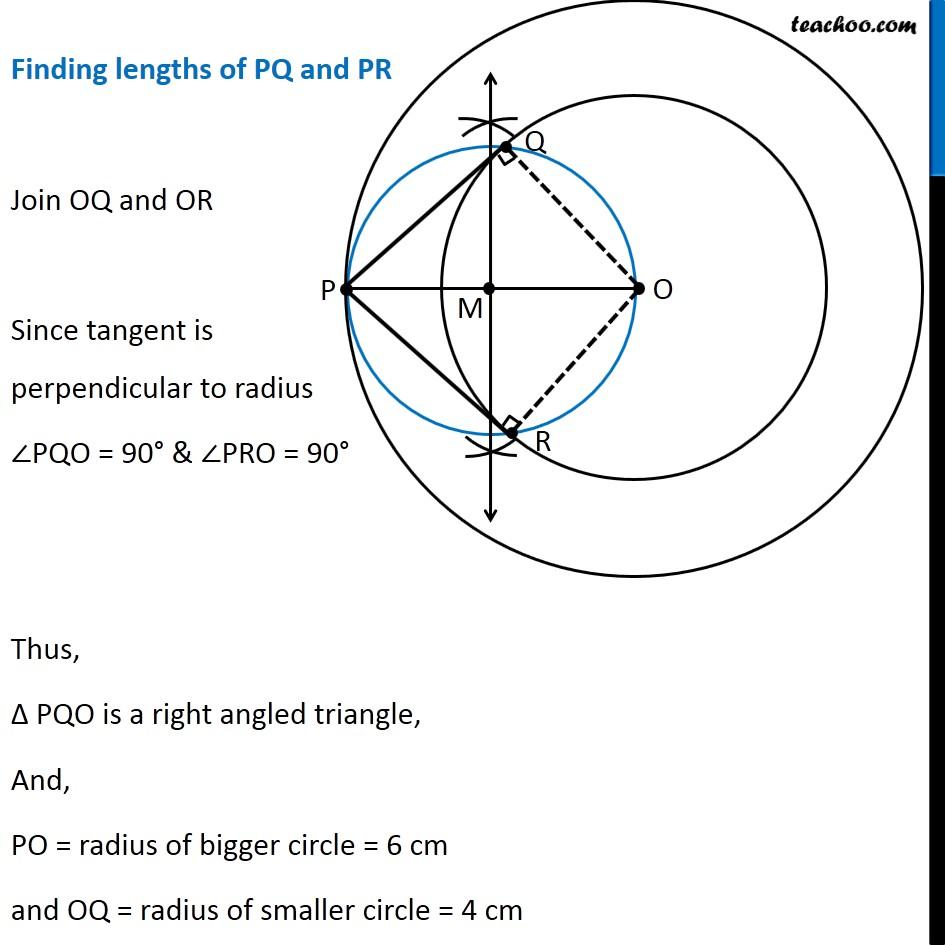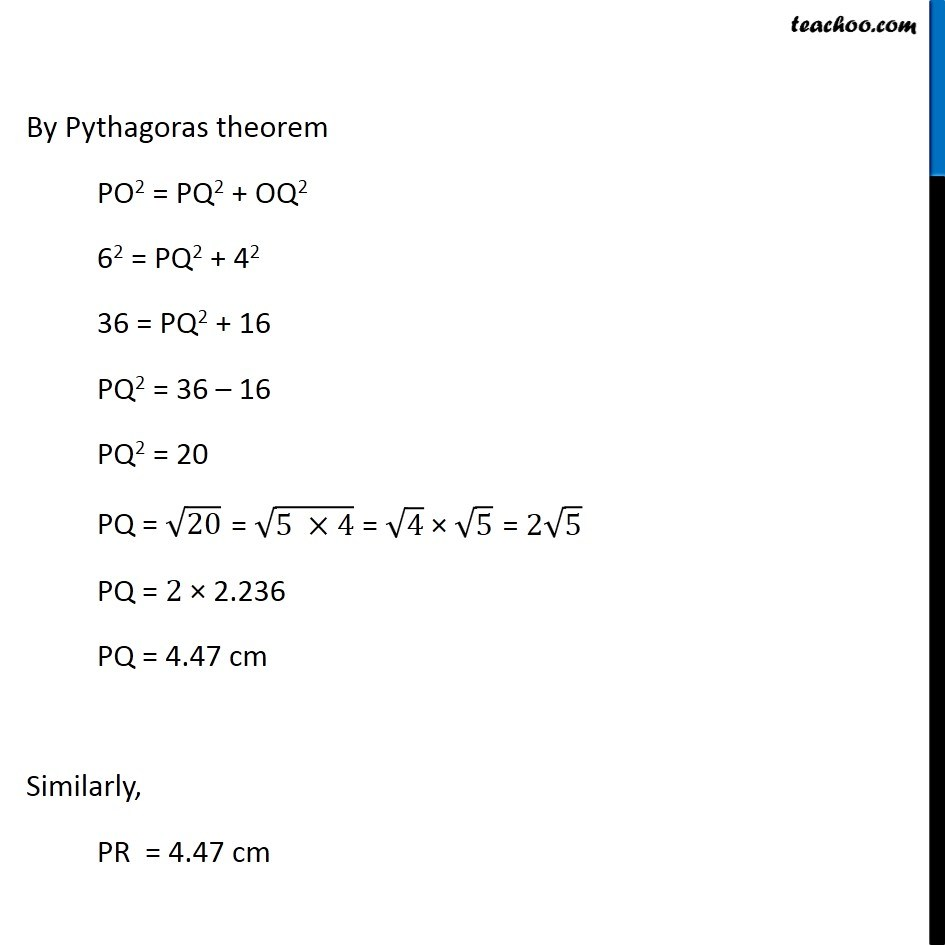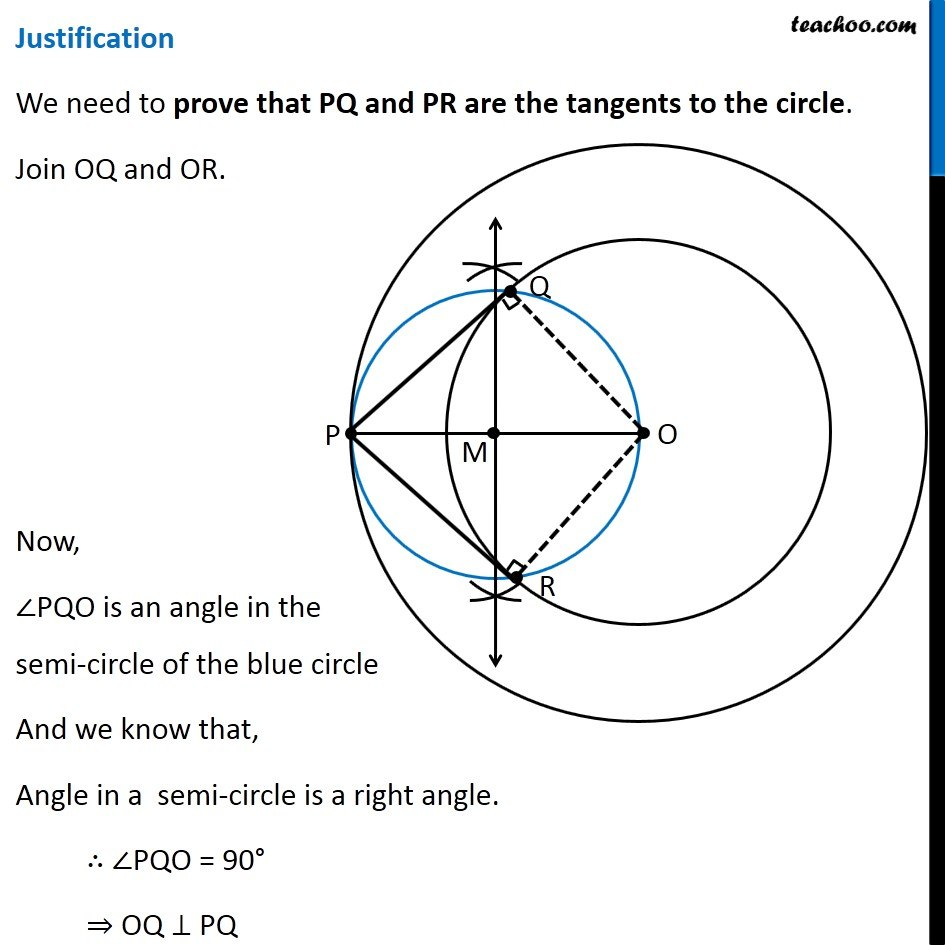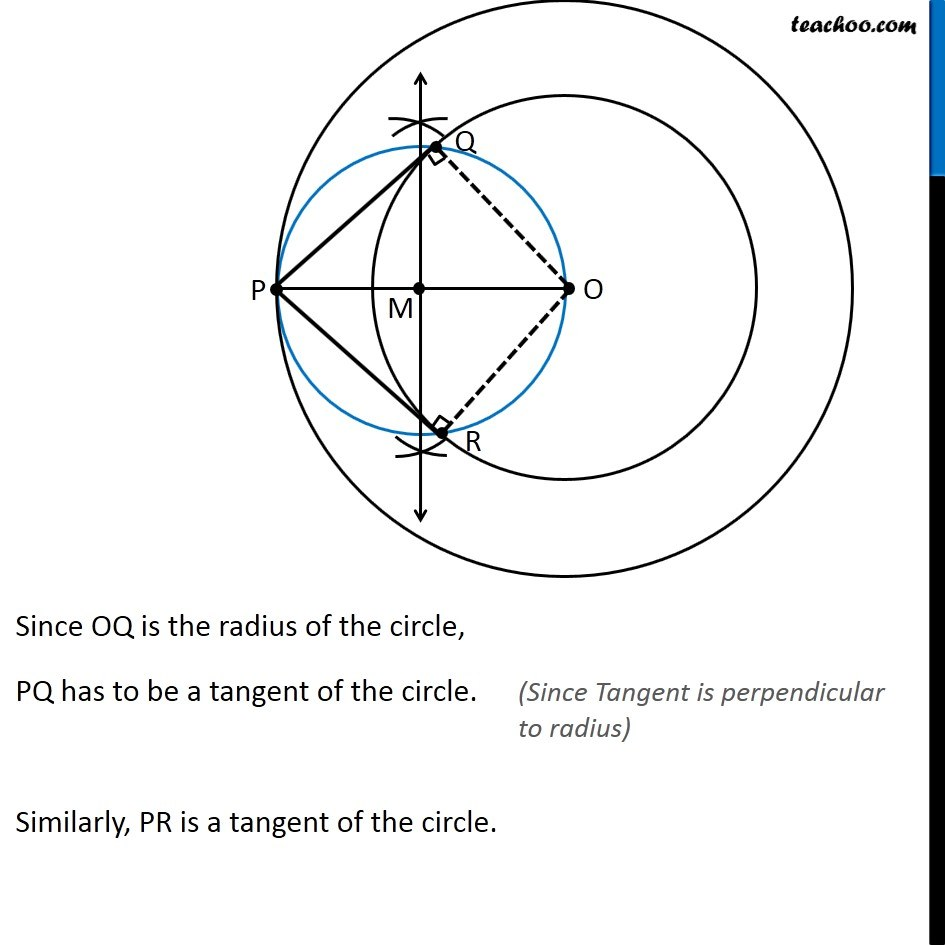1. Chapter 11 Class 10 Constructions
2. Serial order wise
3. Ex 11.2

Transcript

Ex 11.2, 2 Construct a tangent to a circle of radius 4 cm from a point on the concentric circle of radius 6 cm and measure its length. Also verify the measurement by actual calculation. Steps of construction Draw a circle of radius 4 cm with center O Draw concentric circle of radius 6 cm, with center O Mark point P on the larger circle 3. Join PO. Make perpendicular bisector of PO Let M be the midpoint of PO. 4. Taking M as centre and MO as radius, draw a circle. 5. Let it intersect the given circle at points Q and R. 6. Join PQ and PR. ∴ PQ and PR are the required two tangents. After measuring, lengths of tangents PQ and PR are 4.47 cm each Finding lengths of PQ and PR Join OQ and OR Since tangent is perpendicular to radius ∠PQO = 90° & ∠PRO = 90° Thus, Δ PQO is a right angled triangle, And, PO = radius of bigger circle = 6 cm and OQ = radius of smaller circle = 4 cm By Pythagoras theorem PO2 = PQ2 + OQ2 62 = PQ2 + 42 36 = PQ2 + 16 PQ2 = 36 – 16 PQ2 = 20 = √(5 ×4) √4 × √5 = 2√5 PQ = √20 PQ = 2 × 2.236 PQ = 4.47 cm Similarly, PR = 4.47 cm Justification We need to prove that PQ and PR are the tangents to the circle. Join OQ and OR. Now, ∠PQO is an angle in the semi-circle of the blue circle And we know that, Angle in a semi-circle is a right angle. ∴ ∠PQO = 90° ⇒ OQ ⊥ PQ Since OQ is the radius of the circle, PQ has to be a tangent of the circle. (Since Tangent is perpendicular to radius) Similarly, PR is a tangent of the circle.

Ex 11.2